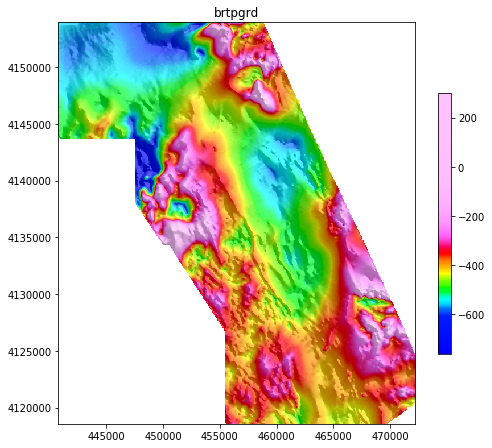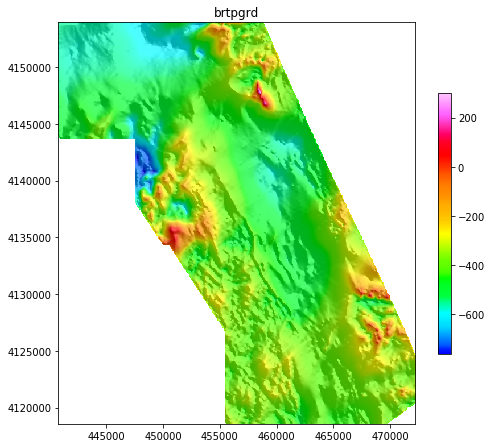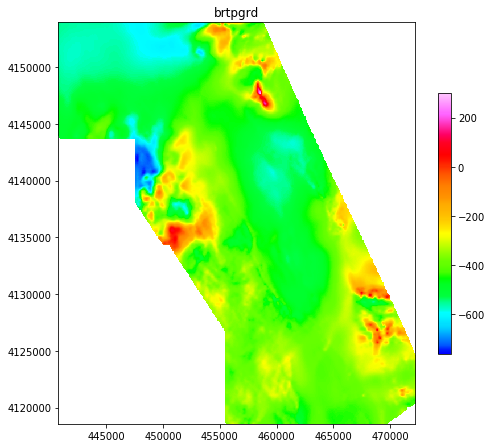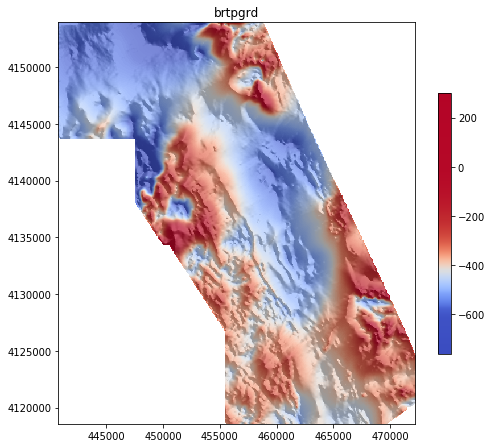# Displaying grids with interpies¶

Joseph Barraud

This notebook introduces the various possibilities of grid display with interpies. The function show combines several functions of matplotlib so that relatively complex maps can be created with only one line of code.

### How to display an uneven data distribution?¶

The range of values of geophysical data, typically from gravity and magnetic surveys, is often dominated by extreme and localised anomalies. Displaying this sort of data can be a challenge. For example, using a linear scale would achieve a true representation of the data content; and large highs and lows would be clearly visible. However, smaller anomalies would also certainly disappear in a large extent of uniform colour.

Two of the most common ways to solve this problem are hillshading, and data normalisation. Hillshading works with derivatives so will naturally remove large trends and highlight small gradients. Normalisation modifies the data to rebalance the distribution of anomalies: the simplest way to do that is to clip highs and lows to reduce the range of values. There are more sophisticated ways to normalise the data, for example using a logarithmic scale. See the matplotlib documentation for more information.

### Colormapping¶

The way data values are turned into colours is called colormapping. As explained in the matplotlib documentation, this involves two steps: (1) the data is normalised to the 0-1 interval, (2) the 0-1 interval is mapped to a sequence of colours (the "colormap"). In this approach, normalisation happens in the first step, and the second step is purely a linear match between two sequences.

In interpies, I have experimented a different approach: instead of modifying the data, the colormap is modified to visually achieve the same result as in the first approach. The two steps of colormapping remain linear but the colormap has changed, for example to rebalance an uneven data distribution.

The advantage is that the colorbar works as a visual indicator of the amount of normalisation that has been applied to the data.

Let's look at a few examples of how this works in practice.

In :
import sys
import numpy as np
import matplotlib.pyplot as plt
% matplotlib inline

In :
import interpies


## Display a grid with default settings¶

The example data come from an aeromagnetic survey collected for the USGS over the town of Blanca in Colorado (Bankey and Grauch, 2004). The survey lines, the gridded data and the report are available from this page.

The grid can be loaded with the function open.

In :
inFile = '../data/brtpgrd.gxf'
grid1 = interpies.open(inFile)


Once loaded in interpies as a Grid object, gridded data can be displayed with the show() method.

In :
ax = grid1.show()The default parameters are the ones from the imshow_hs function in the graphics module.

imshow_hs(source, ax=None, cmap='geosoft', cmap_norm='equalize', hs=True,
zf=10, azdeg=45, altdeg=45, dx=1, dy=1, hs_contrast=1.5, cmap_brightness=1.0,
blend_mode='alpha', alpha=0.7, contours=False, colorbar=True,
cb_contours=False, cb_ticks='linear', std_range=1, figsize=(8, 8),
title=None, **kwargs)

The default normalisation is equalisation. It can have quite a pronounced effect on the result but it is generally well suited for grids with an uneven data distribution. After all, that is what the algorithm of histogram equalisation is about: redistributing values to obtain a flat histogram, therefore maximising the contrast.

## Use a linear scale¶

To understand the impact of equalisation, let's revert to a linear scale, i.e. no normalisation:

In :
ax = grid1.show(cmap_norm='none')Notice how the colorbar has changed between the two plots. The second one is the way it would normally look like with the pyplot.imshow() function. In the previous plot, the colorbar clearly indicates that a large part of the data range is represented by only one colour, so it's an honest way to remind the reader that the colours have been "manipulated" somehow to highlight some of the smaller anomalies.

Now, removing the hillshade demonstrates how useful it is to emphasize small variations in the middle of the range:

In :
ax = grid1.show(cmap_norm='none', hs=False)## Change the colormap¶

...keeping everything else to default values.

In :
ax = grid1.show(cmap='coolwarm')Here is parula, the MATLAB default.

In :
ax = grid1.show(cmap='parula')